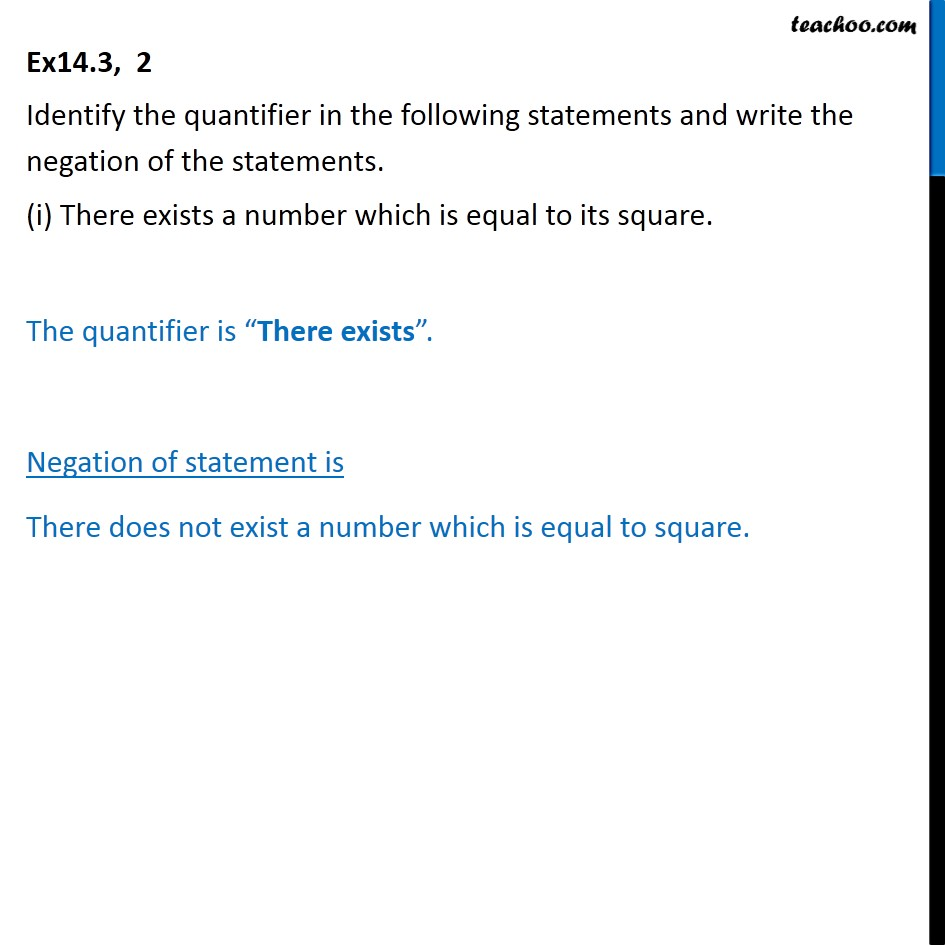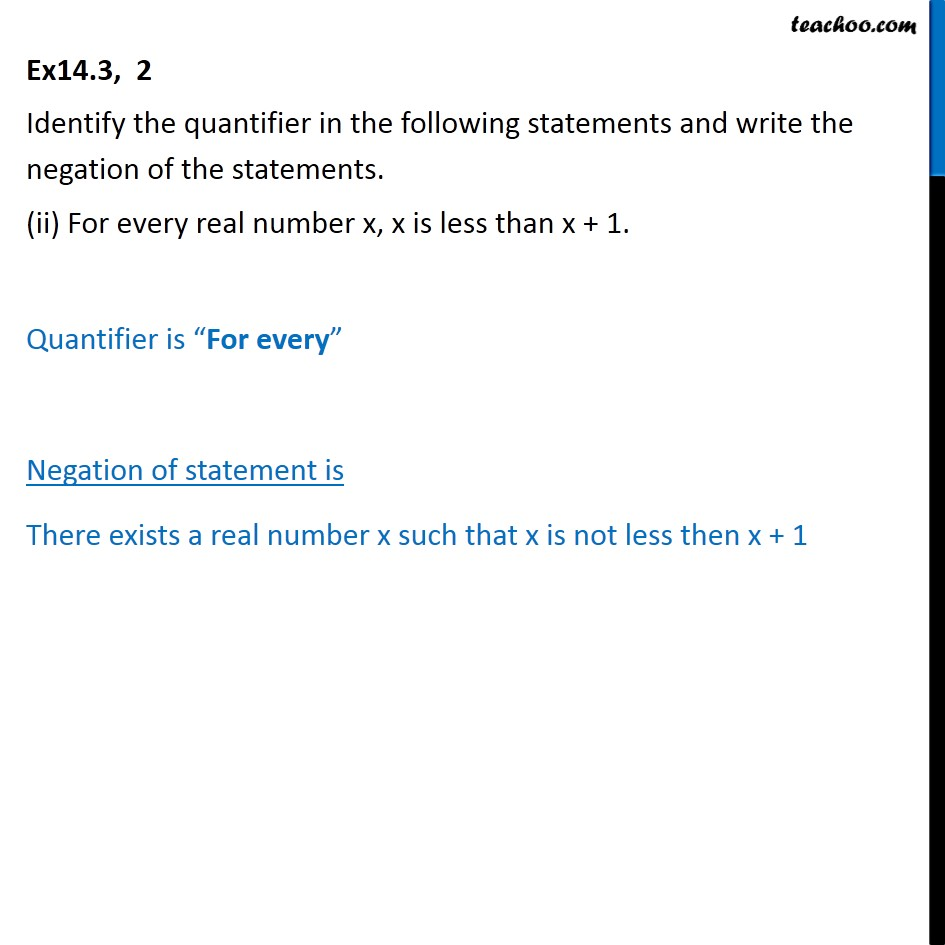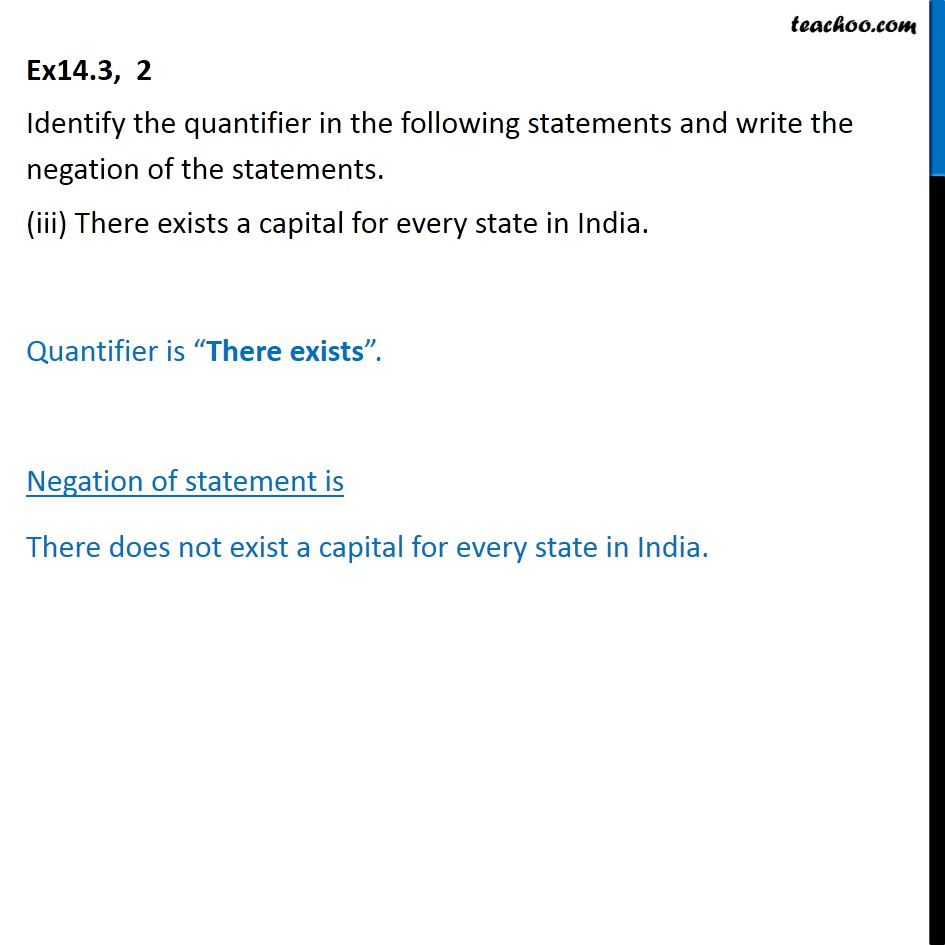Subscribe to our Youtube Channel - https://you.tube/teachoo

1. Chapter 14 Class 11 Mathematical Reasoning
2. Concept wise

Transcript

Ex 14.3, 2 Identify the quantifier in the following statements and write the negation of the statements. (i) There exists a number which is equal to its square. The quantifier is “There exists”. Negation of statement is There does not exist a number which is equal to square. Ex14.3, 2 Identify the quantifier in the following statements and write the negation of the statements. (ii) For every real number x, x is less than x + 1. Quantifier is “For every” Negation of statement is There exists a real number x such that x is not less then x + 1 Ex14.3, 2 Identify the quantifier in the following statements and write the negation of the statements. (iii) There exists a capital for every state in India. Quantifier is “There exists”. Negation of statement is There does not exist a capital for every state in India.